# How to Model ViscoplasticityJanuary 2, 2015 By: Michael Bak

In engineering applications where materials are expected to yield and accumulate plastic deformation, it is typically assumed that the rate of loading does not have an appreciable effect on the material behavior of the structure, i.e. whether we load it quickly or slowly, the material plastic response will be identical.  However, there are many cases in which the material response depends on the rate of the loading, or stated another way, on the strain rate of the material, such as in metal-forming.  This time-dependent response of materials is referred to as rate-dependent plasticity, or viscoplasticity.

There are several material laws that are typically used to model viscoplasticity in a finite element analysis.  Each of these laws represents a different approach and is typically used in different applications:

1) Creep.
2) Peirce or Perzyna.
3) Anand.

A previous blog I wrote recently discussed creep. From a material standpoint, viscoplasticity and creep are the same.  In engineering terms, creep is typically used to describe rate-dependent inelastic behavior assuming the time period is relatively long (in years), and the creep and plastic strains are decoupled, i.e. the rate-dependent creep and rate-independent plastic strains are calculated separately and summed to obtain the total strain.  The separate strain components are typically available in the results (in ANSYS, EPPL contains the plastic strain, and EPCR contains the creep strain).

The Peirce or Perzyna models adopt a unified creep approach to calculate rate-dependent inelastic strain, i.e. creep and plasticity are not calculated separately but instead an inelastic strain is calculated directly.  These models assume that the plastic strain rate is a function of a hardening parameter (m), the material viscosity parameter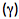, and the static yield stress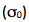. For example, the Perzyna form is shown below: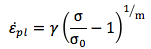These viscoplastic models are suitable for high-strain rate loading situations, such as impact, assumed to cover a much smaller time period than creep.  The Perzyna formula above is identical to the Cowper-Symonds viscoplastic formula that was presented in a previous blog post on strain-rate effects in explicit dynamics using codes such as LS-DYNA.  These models include a yield surface; therefore, the strain-rate hardening effect is only active after yielding, and an isotropic hardening plasticity model must be defined and combined with the viscoplastic model.  An illustration of the expected stress-strain response of a Perzyna model with changing strain rate is shown below.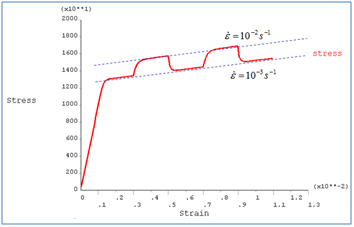Note that plastic (rate-independent) and viscoplastic (rate-dependent) strains are coupled and not separable.  The output of total inelastic strain is provided (in ANSYS via the plastic strain EPPL).  Also, when the viscosity tends to infinity, the strain rate tends to zero, and the solution converges to the rate-independent solution, as expected.

Anand's model is used in cases where the material behavior is very sensitive to strain rate, temperature, history of strain rate and temperature, and strain hardening and softening.  Anand's model was originally developed for high-temperature metal forming processes such as rolling and deep-drawing, but it has been demonstrated for use in predicting the response and life of solder joints in electronic packaging. CAE Associates has used both the creep approach and Anand's model to predict the life of solder joints in our FEA consulting projects.

This model introduces a single scalar quantity called the deformation resistance (s), which is used to represent the isotropic resistance to inelastic flow of the material.  The equation for inelastic strain rate is given below: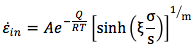Note that this equation has the same basic form as the Generalized Garofalo creep equation, except that instead of a constant term (C2), Anand contains the deformation resistance term (s), which is time-dependent.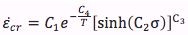Anand's model requires nine (9) different material constants, found by curve-fitting a series of isothermal stress-strain tensile tests at different temperatures and strain rates.  Anand material constants for various solder materials are available in the literature. There is no explicit yield surface, plastic flow is assumed to occur at all non-zero stress values, and no hardening law is required. This viscoplastic law assumes a unified approach, thus the inelastic strain is returned (in ANSYS via the plastic strain EPPL).  In addition, the deformation resistance can typically be obtained with the results, as well as the plastic work per volume. This last term can be used for fatigue calculations, since relationships exist for various solder materials that describe the amount of accumulated plastic work that will cause failure. By determining the plastic work accumulated in one cycle, the total number of cycles can be determined.

In summary, there are several ways to incorporate the effect of rate-dependent inelastic response in finite element analyses. The choice of the material model to use is based on the expected response of the structure and the material data available. If you have used any of these methods, or if you have comments or questions, we would love to hear from you.